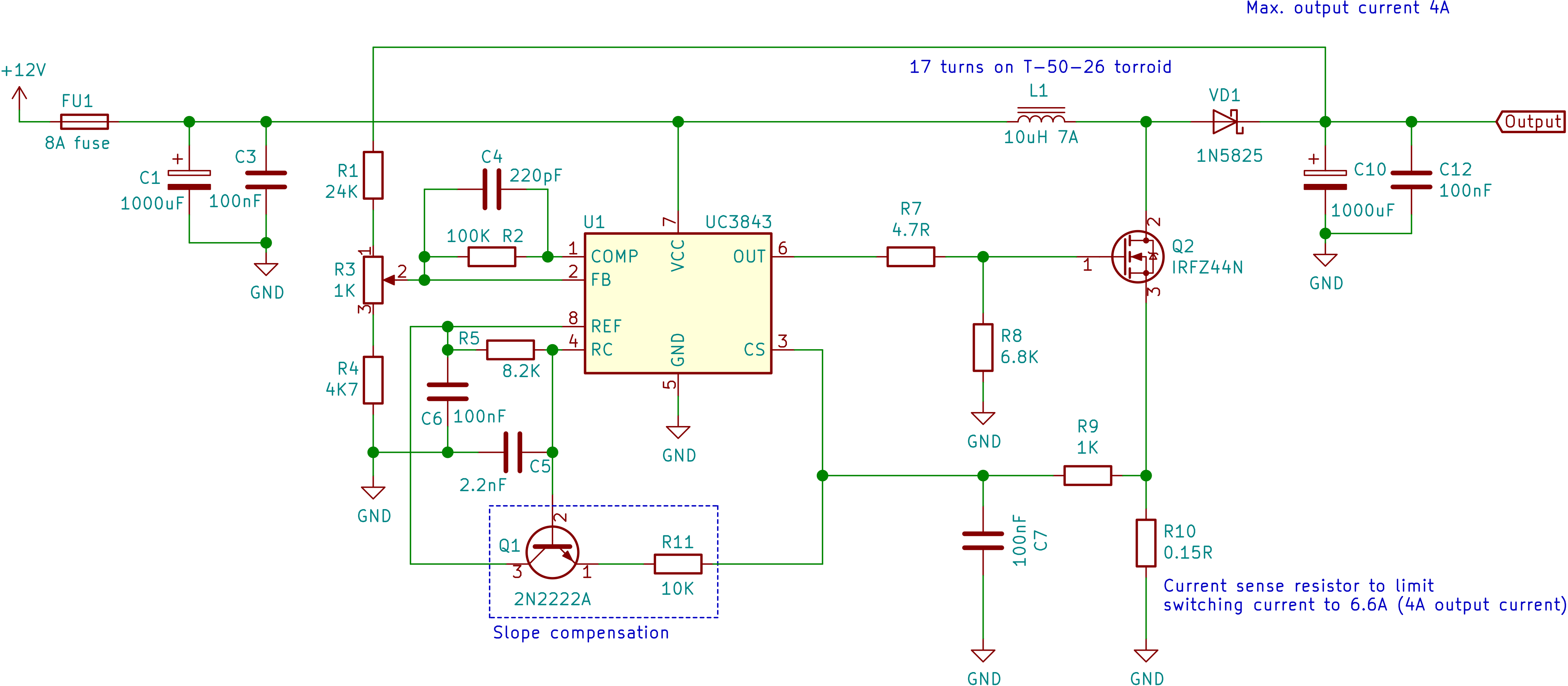# Fixed Output Boost Converter Using UC3843¶

Design boost converter to get output voltage 14.2 (to charge lead-acid batteries) or other nearby voltages by adjusting resistor divider, from voltage source around 12V.

It is based on document Basic Calculation of a Boost Converter's Power Stage. Also read UC3843 datasheet from Texas Instruments, which has lots of useful information for designs around UC3843 IC.

Here is circuit diagram. Below I will try to explain functions of most of the parts used in it.## Parameters¶

• Minimum input voltage, VIN(min) = 11.5 V
• Maximum input voltage, VIN(max) = 12.5 V
• Average input voltage, VIN = 12 V
• Output voltage, VOUT = 14.2 V
• Maximum output current, IOUT = 4 A
• Expected efficiency, η = 80%
• Frequency, f = 100 kHz
• Forward voltage of the rectifier diode, VF = 0.75 (of 1N5825)

## Inductor Selection¶

Inductance,

Thus inductance, L = 9.8 µH

## Calculate Maximum Switching Current¶

Duty cycle,

Thus, D = 35.21%

Inductor ripple current,

Inductor ripple current, ΔIL = 1.89 A

Maximum switching current,

Thus, ISW(max) = 7.1 A

## Rectifier Diode Selection¶

To reduce losses,Schottky diodes should be used.The forward current rating needed is equal to the maximum output current:

IF = IOUT(max)

Maximum average rectified forward current of 1N5825 is 5 A. So it is within the limits

Power dissipation of the diode,

Thus PD = 3 W

## Resistive Divider for Output Voltage Setting¶

Feedback voltage for UC3843 is 2.5 V.

Current through the resistive divider should be at least 100 times as big as the feedback bias current.

So,

Info

Feedback input bias current of UC3843 taken as 2 µA. That value is taken from datasheet of UC3843 from On Semiconductor

So, R2 = 12.5 kΩ

To find R1, equation is

Thus R1 = 58.5 kΩ

## Output Capacitor Selection¶

Desired output ripple voltage ΔVOUT = 0.1 V

Minimum output capacitance

Thus COUT(min) = 140.8 µF

However using larger capacitance will be good. Larger capacitance will reduce ripple voltage and ESR because of larger plates.

## PCB Layout¶